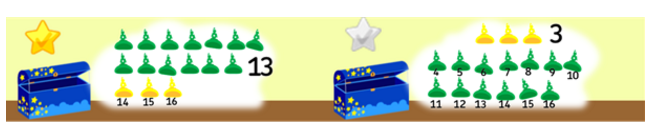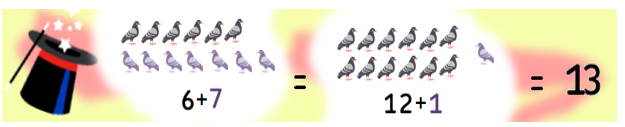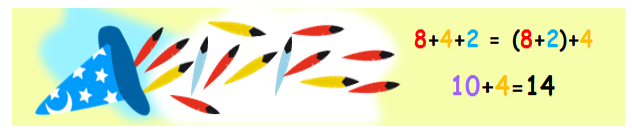Smartick is an advanced online program that teaches kids math and coding in only 15 min. a day

Feb08

# Learn 5 Tricks for Addition and Some Examples

### 1. Count from the number

For example: 7 + 2• Start on 7
• Count 2 more…until you hit 9
• So, 7 + 2 = 9

For example: 3 + 13

If I have 3 yellow hats and 13 green hats inside of my trunk, and I ask you how many hats I have all together…It’s easier to start from the number 13 and add 3 than it would be to do it the other way around. 13 + 3 = 16

### 3. Multiply by 2

Sometimes finding the double can save you a lot of time.

For example: 17 + 19

• Find the number that is in between the numbers you need to add, 17, 18 , 19
•  Multiply it by 2: 18 x 2 = 36
• And there you have it, 17 + 19 = 36

And if the numbers you need to add are consecutive, multiply the smaller number by two and add on the other one.

For example: 6 + 7

If I take out 6 grey doves and 7 purple ones from my top hat, and I ask you how many doves there are in total…

6 and 7 are consecutive numbers, so you can use this trick:

• Multiply the smaller number by two, which in this case is 6.

6 x 2 = 12

• Add on 1 to the answer of that multiplication. 12 + 1 = 13
• Now you know that 6 + 7 = 13### 4. Combine numbers to form groups of 10

When you have to add more than 2 numbers, whenever you can, combine the numbers that can give you 10.

For example: If you want to know how many total feathers flew out from the hat, you have to add

8 red feathers + 4 yellow feathers + 2 blue feathersTo make it a little easier:

• Find the numbers that add up exactly to 10:  8+2=10
• Add on the rest of the numbers: 10+4=14

### 5. Find the group of 10s

When one of the numbers that you need to add is close to 10, round it off to 10 and add or subtract whatever the number you used to make it 10.

For example: 7 + 9

• Substitute the 9 with (10-1) and you’ll get 7 + (10 – 1)
• Add 7 +10 = 17
•  …and now you just need to subtract 1: 17-1=16Did you like this post? Share it with your friends so they can see these adding tricks, too.

Check out more addition posts on Smartick below:

If you want to practice these kinds of problems, and more, go to Smartick and try it for free!